##### The Japanese Bonsai specialist
Direct order Contact Help / Services Newsletter# Brass watering spout 600 mm and nozzle

› Bonsai tools › Watering equipment › Watering nozzles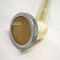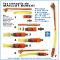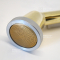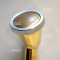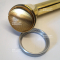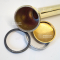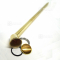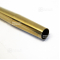ref. : 10979

48,00

Available quantity : 4Order

###### Description

Maillot Bonsai has used this watering can brass copper spout and nozzle for years. It’s indispensable if you have outdoor bonsai, which adore the fine rain it pours. Ø of the rosette 50 mm. Removable grid, length: 345 mm. If you connect it to a garden hose or watering system you can conveniently adjust the tap to regulate the flow.

#watering 6.8 #nozzle 3.6 #brass 3.5 #spout 3.5 #nozzles 2.7 #bonsai 2.6 #indispensable 2.3 #conveniently 2.2 #removable 1.9 #regulate 1.8

Formule
(( ROUND((CHAR_LENGTH(b.article_nom)-CHAR_LENGTH(REPLACE(b.article_nom, 'watering', '')))/LENGTH('watering')) + ROUND((CHAR_LENGTH(b.article_description)-CHAR_LENGTH(REPLACE(b.article_description, 'watering', '')))/LENGTH('watering')) ) * 4.8) + (( ROUND((CHAR_LENGTH(b.article_nom)-CHAR_LENGTH(REPLACE(b.article_nom, 'nozzle', '')))/LENGTH('nozzle')) + ROUND((CHAR_LENGTH(b.article_description)-CHAR_LENGTH(REPLACE(b.article_description, 'nozzle', '')))/LENGTH('nozzle')) ) * 3.6) + (( ROUND((CHAR_LENGTH(b.article_nom)-CHAR_LENGTH(REPLACE(b.article_nom, 'brass', '')))/LENGTH('brass')) + ROUND((CHAR_LENGTH(b.article_description)-CHAR_LENGTH(REPLACE(b.article_description, 'brass', '')))/LENGTH('brass')) ) * 3.5) + (( ROUND((CHAR_LENGTH(b.article_nom)-CHAR_LENGTH(REPLACE(b.article_nom, 'spout', '')))/LENGTH('spout')) + ROUND((CHAR_LENGTH(b.article_description)-CHAR_LENGTH(REPLACE(b.article_description, 'spout', '')))/LENGTH('spout')) ) * 3.5) + (( ROUND((CHAR_LENGTH(b.article_nom)-CHAR_LENGTH(REPLACE(b.article_nom, 'bonsai', '')))/LENGTH('bonsai')) + ROUND((CHAR_LENGTH(b.article_description)-CHAR_LENGTH(REPLACE(b.article_description, 'bonsai', '')))/LENGTH('bonsai')) ) * 2.6) + (( ROUND((CHAR_LENGTH(b.article_nom)-CHAR_LENGTH(REPLACE(b.article_nom, 'indispensable', '')))/LENGTH('indispensable')) + ROUND((CHAR_LENGTH(b.article_description)-CHAR_LENGTH(REPLACE(b.article_description, 'indispensable', '')))/LENGTH('indispensable')) ) * 2.3) + (( ROUND((CHAR_LENGTH(b.article_nom)-CHAR_LENGTH(REPLACE(b.article_nom, 'conveniently', '')))/LENGTH('conveniently')) + ROUND((CHAR_LENGTH(b.article_description)-CHAR_LENGTH(REPLACE(b.article_description, 'conveniently', '')))/LENGTH('conveniently')) ) * 2.2) + (( ROUND((CHAR_LENGTH(b.article_nom)-CHAR_LENGTH(REPLACE(b.article_nom, 'removable', '')))/LENGTH('removable')) + ROUND((CHAR_LENGTH(b.article_description)-CHAR_LENGTH(REPLACE(b.article_description, 'removable', '')))/LENGTH('removable')) ) * 1.9) + (( ROUND((CHAR_LENGTH(b.article_nom)-CHAR_LENGTH(REPLACE(b.article_nom, 'regulate', '')))/LENGTH('regulate')) + ROUND((CHAR_LENGTH(b.article_description)-CHAR_LENGTH(REPLACE(b.article_description, 'regulate', '')))/LENGTH('regulate')) ) * 1.8) + (( ROUND((CHAR_LENGTH(b.article_nom)-CHAR_LENGTH(REPLACE(b.article_nom, 'maillot', '')))/LENGTH('maillot')) + ROUND((CHAR_LENGTH(b.article_description)-CHAR_LENGTH(REPLACE(b.article_description, 'maillot', '')))/LENGTH('maillot')) ) * 1.7)

## Secure payment## Delivery

Our logistic partners :04 74 55 23 48
Pépinière MAILLOT-BONSAÏ
Le Bois Frazy
01990 RELEVANT - FRANCE
on appointment